## The exceptional Jordan algebra

In the early 1930s, Pascual Jordan attempted to formalise the algebraic properties of Hermitian matrices. In particular:

• Hermitian matrices form a real vector space: we can add and subtract Hermitian matrices, and multiply them by real scalars. That is to say, if$\lambda, \mu \in \mathbb{R}$ and$A, B$ are Hermitian matrices, then so is the linear combination$\lambda A + \mu B$.
• We cannot multiply Hermitian matrices and obtain a Hermitian result, unless the matrices commute. So the matrix product$AB$ is not necessarily Hermitian, but the ‘symmetrised’ product$A \circ B = \frac{1}{2}(AB + BA)$ is Hermitian, and coincides with ordinary multiplication whenever the matrices commute.

Now, this symmetrised product$A \circ B$ is commutative by definition, and is also (bi)linear:$(\lambda A + \mu B) \circ C = \lambda (A \circ C) + \mu (B \circ C)$. What other algebraic properties must this product satisfy? The important ones are:

• Power-associativity: the expression$A^n = A \circ \cdots \circ A$ does not depend on the parenthesisation.
• Formal reality: a sum of squares is zero if and only if all of the summands are zero.

The second of these conditions means that we can say that an element of the Jordan algebra is ‘nonnegative’ if it can be expressed as a sum of squares. (In the familiar context of real symmetric matrices, this coincides with the property of the matrix being positive-semidefinite.) The nonnegative elements form a ‘cone’ closed under multiplication by positive real scalars and addition.

Jordan, von Neumann, and Wigner proceeded to classify all of the finite-dimensional algebras of this form (known as formally real Jordan algebras). They showed that every such algebra is a direct sum of ‘simple’ algebras, each of which is isomorphic to [at least] one of the following:

• the real symmetric matrices of dimension n (for any positive integer n) with the aforementioned symmetrised product;
• the complex Hermitian matrices of dimension n;
• the quaternionic Hermitian matrices of dimension n;
• the octonionic Hermitian matrices of dimension n (where n ≤ 3);
• the algebras$\mathbb{R}^n \oplus \mathbb{R}$ with the product$(x, t) \circ (x', t') = (t'x + tx', \langle x, x' \rangle + tt')$, known as ‘spin factors’. As John Baez mentions, these can be identified with Minkowski space, and the nonnegative elements are exactly the ‘future cone’ of the origin.

The qualification ‘at least’ is because there are some isomorphisms here: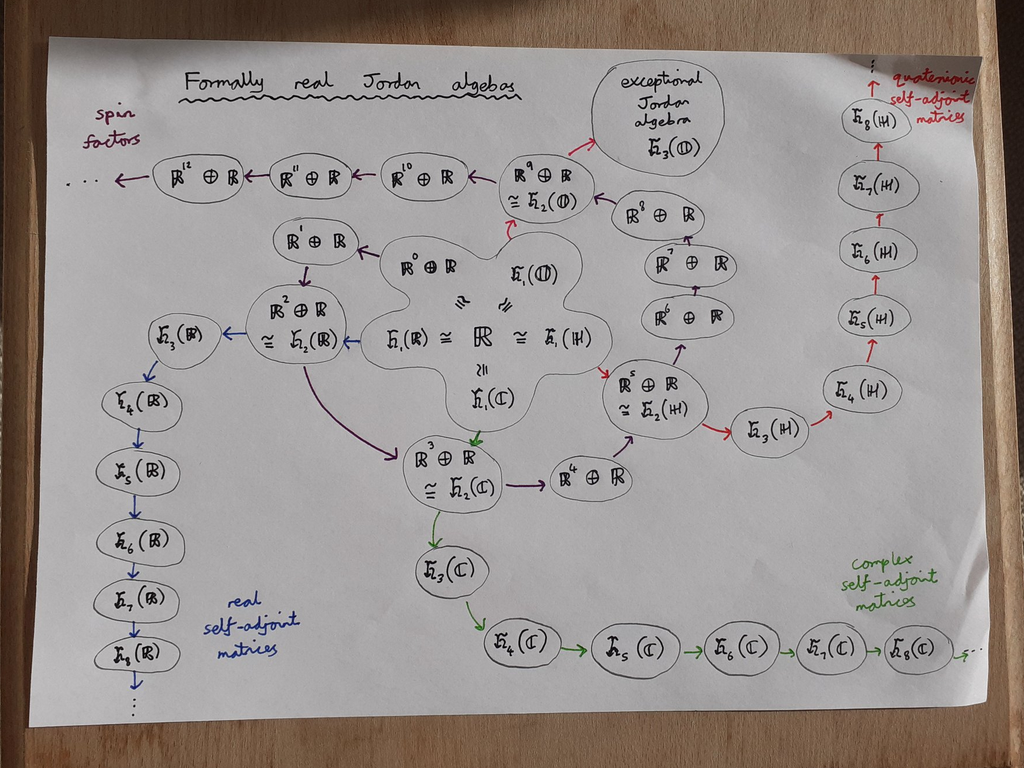Simple formally real Jordan algebras, showing the four infinite families and the exceptional Jordan algebra

Exactly one of these simple formally real Jordan algebras fails to fit into any of the four infinite families. This exceptional Jordan algebra is$\mathfrak{h}_3(\mathbb{O})$, the 3-by-3 self-adjoint octonionic matrices endowed with the symmetrised product. Viewed as a real vector space, it is 27-dimensional: an arbitrary element can be described uniquely by specifying the three diagonal elements (which must be real) and three lower off-diagonal elements (which can be arbitrary octonions); the three upper off-diagonal elements are then determined.

### Projective spaces from Jordan algebras

Given a formally real Jordan algebra, we can consider the idempotent elements satisfying$A \circ A = A$. For the Jordan algebras built from n-by-n real, complex, or quaternionic matrices, these are the matrices with eigenvalues 0 and 1.

We get a partial order on these ‘projection’ matrices: A ‘contains’ B if and only if$A \circ B = B$. This partially-ordered set can be identified with the stratified collection of subspaces in the (n−1)-dimensional projective space over the base field:

• the zero matrix corresponds to the empty space;
• the rank-1 projection matrices correspond to points;
• the rank-2 projection matrices correspond to lines;
• the rank-(n−1) projection matrices correspond to hyperplanes;
• the identity matrix corresponds to the full projective space itself.

The exceptional Jordan algebra gives us the octonionic projective plane, discovered by Ruth Moufang. We can’t get any higher-dimensional octonionic projective spaces because Desargues’ theorem is false in the octonionic projective plane, whereas it’s true in any plane that can be embedded in a 3-dimensional projective space. We mentioned this seven years ago.

This hints at why 4-by-4 and higher octonionic matrices have no hope of forming a formally real Jordan algebra: we’d be able to define an octonionic projective 3-space, which is impossible.

What about the spin factors? The idempotents in$\mathbb{R}^n \oplus \mathbb{R}$ are:

• the zero element (0, 0), corresponding to the ’empty space’;
• the identity element (0, 1), corresponding to the ‘full space’;
• the points (x, ½) where x is an arbitrary vector of length ½.

In other words, these correspond to spheres! Recall that the real, complex, quaternionic, and octonionic projective lines are (as topological manifolds) just the 1-, 2-, 4-, and 8-spheres, respectively; we can think of the spin factors as ‘projective lines’ built from arbitrary-dimensional spheres.

As for non-simple formally real Jordan algebras, the corresponding ‘projective spaces’ are just Cartesian products of the ‘projective spaces’ corresponding to the direct summands.

### An exotic spacetime

In August of this year, Blake Stacey posted the following comment on John Baez’s essay: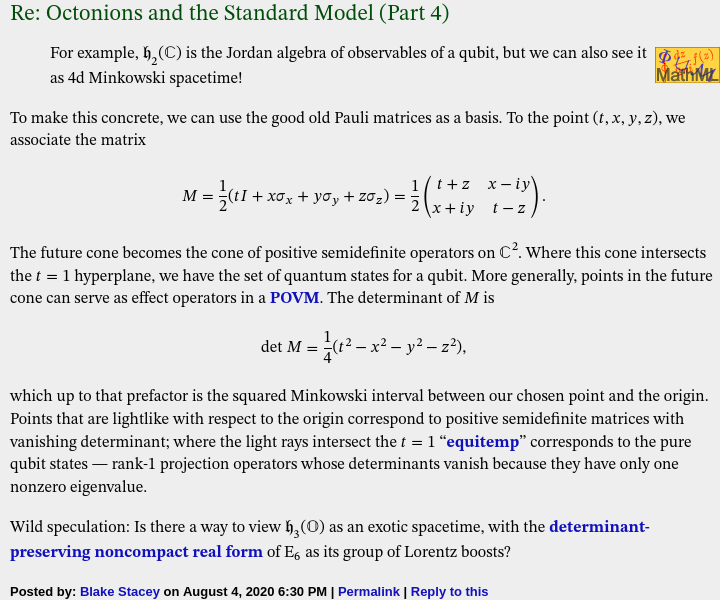Now for some context: it is possible to define the determinant of a 3-by-3 octonionic Hermitian matrix, and the group of linear operators (viewing$\mathfrak{h}_3(\mathbb{O})$ as a 27-dimensional real vector space) that preserves the determinant is a noncompact real form of the Lie group E6.

This group E6 is transitive on the positive-definite matrices of determinant 1. The subgroup fixing any one of these (without loss of generality, the identity matrix) is the compact real Lie group F4, which also preserves the Jordan product. This means that it maps idempotents to idempotents, so can be seen as acting on the octonionic projective plane as its group of projective transformations.

This group F4 is transitive on the rank-1 idempotent matrices, and the subgroup fixing any one of these is Spin(9). (As a result, we can describe the octonionic projective plane as the quotient F4 / Spin(9). Elie Cartan proved that all compact Riemannian symmetric spaces are quotients of compact Lie groups.)

What’s the analogy for familiar (3+1)-dimensional Minkowski spacetime?

• the full group (analogous to E6) is the proper orthochronous Lorentz group;
• the subgroup fixing a timelike vector (analogous to F4) is the rotation group SO(3);
• the subgroup additionally fixing a lightlike vector (analogous to Spin(9)) is the rotation group SO(2);
• the symmetric space (analogous to the octonionic projective plane) is the quotient SO(3) / SO(2), which is just the familiar 2-sphere.

### A lattice in this exotic spacetime

It is natural to consider the ‘integer points’ in this spacetime, namely the octonionic Hermitian matrices where the off-diagonal elements are Cayley integers and the diagonal elements are ordinary integers. John Baez mentions that this is the unique integral unimodular lattice in (26+1)-dimensional spacetime, and it can be seen as the direct sum$II_{25,1} \oplus \mathbb{Z}$ of the exceptional Lorentzian lattice with a copy of the integers.

This lattice was thoroughly investigated in a marvellous paper by Noam Elkies and Benedict Gross. Possibly the most surprising discovery in this paper is that whilst E6 acts transitively on the positive-definite matrices of determinant 1, this no longer holds when you ‘discretise’! More precisely, the positive-definite ‘integer points’ of determinant 1 form two distinct orbits under the discrete subgroup of E6 that preserves the lattice.

One of these orbits contains the identity matrix; the other contains the circulant matrix with elements {2, η, η*} where$\eta = \dfrac{-1 + \sqrt{-7}}{2}$. [Note: there’s a 6-dimensional sphere of octonionic square-roots of -7. You’ll need to choose one that results in η being a Cayley integer.] If you use this other matrix E as your quadratic form instead of the identity matrix I, this leads to a very natural construction of the Leech lattice.

Specifically, as shown in the Elkies-Gross paper, triples of Cayley integers with the norm$\langle x | E | x \rangle$ form an isometric copy of the Leech lattice! By contrast, the usual inner product$\langle x | I | x \rangle$ using the identity matrix as the quadratic form gives the direct sum$E_8 \oplus E_8 \oplus E_8$ — again an even unimodular lattice in 24 dimensions, but not as exceptional or beautiful or efficient as the Leech lattice.

To get a full understanding of the octonions, Cayley integers, and exceptional Jordan algebra, I recommend reading all of the following:

Robert Wilson has also constructed the Leech lattice from the integral octonions (see here and here). Wilson’s construction also involves$\eta = \dfrac{-1 + \sqrt{-7}}{2}$, so it may be possible to show reasonably directly that it’s equivalent to the Elkies-Gross construction.

## Closed-form numbers

Recall that the logarithm function (defined on the punctured complex plane) is multi-valued, with the different solutions to exp(x) = y differing by integer multiples of 2πi.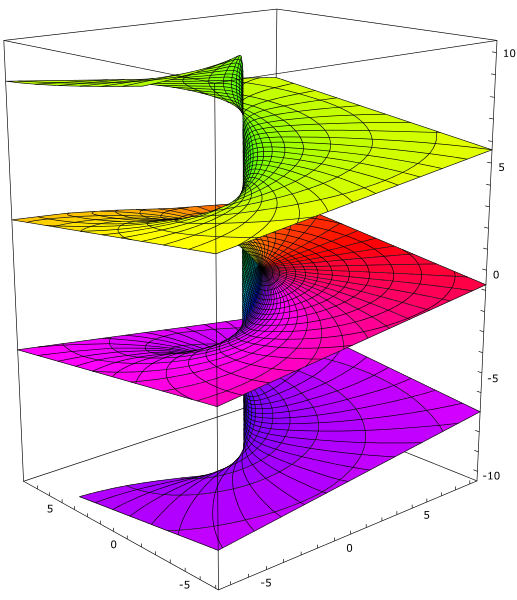Visualisation of the imaginary part of the multi-valued complex logarithm function, created by Leonid 2.

James Propp remarked that the values of the form i^i^i are a dense subset of the unit circle. Extending this, one can show that the values of the form i^i^i^i are not dense in the complex plane, but those of the form i^i^i^i^i are.

### Defining the EL-numbers

Timothy Chow described an interesting subfield of the complex numbers, known as either closed-form numbers or (less ambiguously) EL-numbers. We’ll present a slightly different but equivalent definition here, where we embrace the complex logarithm function in its full multivalued glory instead of choosing a principal value of the logarithm function.

In particular, the EL-numbers can be defined as follows:

• Zero: the complex number 0 is an EL-number;
• Addition/subtraction*: given two EL-numbers, a and b, then f(a, b) is also an EL-number where c = f(a, b) is the solution to a + b + c = 0;
• Exponentiation: given an EL-number x, then exp(x) is also an EL-number;
• Logarithm: given a nonzero EL-number y, then (at least**) one solution of exp(x) = y is also an EL-number.

*this slightly unconventional operation f, which is equivalent to having both addition and subtraction rules, was chosen because it’s completely symmetric: the validity of c = f(a, b) is invariant under permutation of a, b, and c. The relevance of this will become apparent in a future post.

**we show that this implies that all solutions are EL-numbers, so this definition is well-defined.

The first two rules provide us with unary negation and addition, so we know that the EL-numbers form an additive group:

• x = f(x, 0);
• xy = f(f(x, y), 0).

Togther with the other two rules, we can show that the EL-numbers form a field. In particular, if x and y are nonzero, then exp(log(x) + log(y)) = xy and exp(−log(x)) = 1/x irrespective of which choices of logarithms were taken. Finally, we need the multiplicative identity 1 to exist, but that can be obtained straightforwardly as exp(0).

Consequently, every rational number is a deterministic EL-number: we can, for each rational q, write down an expression which evaluates to q irrespective of which choices of logarithms are taken. Other deterministic EL-numbers include e, which can be obtained as exp(exp(0)). More generally, every element of the smallest field containing the rationals and closed under exponentiation is also a deterministic EL-number, and we conjecture that the converse is also true.

### Mitigating multivaluedness

To show that we can obtain all logarithms (not just one), we apply the following procedure:

• Apply the logarithm rule to obtain some solution x to exp(x) = y. This may be different from the ‘desired’ solution, w;
• Apply the logarithm rule to obtain some solution p to exp(p) = −1. This is necessarily some odd integer multiple of πi.
• We know that wx is therefore a rational multiple of p, say qp, so we can construct q deterministically, multiply it by p, and add the result to x to obtain w.

Note that this was somewhat ‘interactive’: we needed to know what solutions x and p were obtained in order to choose the correct rational number q to use. The ‘closed-form expression’ for w depends on the branches of the logarithms taken, and each of these expressions are ‘nondeterministic’ in the sense that if someone changed which branches were taken, they would no longer define w.

### Nondeterministic EL-numbers

Clearly, some deterministic EL-numbers can have nondeterministic expressions: for example, exp(log(exp(0))/2) can be either 1 or −1, even though these are both deterministic EL-numbers. Are there any EL-numbers which only have nondeterministic expressions?

Well, yes: the imaginary unit i can be obtained as exp(log(−1) / 2). We’ll call this a nondeterministic EL-number, because any closed-form expression for i is also a closed-form expression for −i. By the same argument (namely the fact that complex conjugation is an exponential field automorphism of the complex numbers), every nonreal EL-number is necessarily nondeterministic.

π is also an EL-number, because both πi and i have already been shown to be EL-numbers, and we know that they form a field. Is it a deterministic EL-number? Certainly it hasn’t been proved that this is not the case, because it hasn’t even been shown that e + π is irrational. But on the other hand, it seems very difficult to find a construction of π that doesn’t also end up constructing an infinite number of other multiples of π.

My suspicion is that π is not a deterministic EL-number — can this be proved, conditional on a plausible assumption such as Schanuel’s conjecture?

## The Barnes-Wall lattices

For any nonnegative integer n, there exists the highly symmetric Barnes-Wall lattice in dimension$2^n$. In low dimensions, these are (up to scaling and rotation) familiar lattices:

• For n = 0, this is just the integer lattice,$\mathbb{Z}$.
• For n = 1, the lattice is$D_2$, a scaled and rotated copy of the familiar square lattice$\mathbb{Z}^2$.
• For n = 2, we obtain the$D_4$ lattice (consisting of all integer points with even coordinate sum). The convex hull of the vectors closest to the origin form a 24-cell, a self-dual regular polytope in four dimensions.
• For n = 3, this is a scaled copy of the$E_8$ lattice, discovered by Thorold Gosset and proved by Maryna Viazovska to be the densest packing of unit spheres in eight-dimensional space.
• For n = 4, this is a scaled copy of the 16-dimensional laminated lattice,$\Lambda_{16}$, which again is the densest known lattice packing in its dimension. In Conway and Sloane’s Sphere Packings, Lattices and Groups, this is additionally described as a section of the Leech lattice.

We’ll describe a construction of the Barnes-Wall lattices that makes their full symmetry groups apparent (with the unavoidable exception of n = 3). Unlike the other simple constructions of the Barnes-Wall lattices, which involve quadratic fields, this construction only makes use of the classical integers$\mathbb{Z}$.

### The real Clifford group and the minimal vectors

Being slightly notationally liberal (denoting a Weyl group by the name of its Dynkin diagram), let$F_4 \subset O(4)$ be the symmetry group of a standard 24-cell. It has order 1152. In particular, it contains an index-3 subgroup consisting of the 384 signed permutation matrices; the remaining 768 elements are precisely the 4-by-4 Hadamard matrices (see previous post), appropriately scaled so as to be orthogonal matrices.

Being extremely notationally liberal, for n ≥ 3 we let$F_{2^n} \subset O(2^n)$ be generated by:

• the symmetric group$S_n$, which acts naturally on$\mathbb{R}^{2^n}$ when considered as an n-fold tensor product of$\mathbb{R}^2$ with itself;
• the group$F_4$, where we expand each of the$4 \times 4$ matrices to a$2^n \times 2^n$ matrix by taking the Kronecker product with a$2^{n-2} \times 2^{n-2}$ identity matrix.

Equivalently, in the language of minimalistic quantum computation, it’s the group generated by (real orthogonal) 2-qubit gates with dyadic rational entries. (These are exactly elements of F_4 conjugated by elements of S_n.)

Technically, we’ve only defined the groups$F_{2^n}$ for n ≥ 2. For completeness, we define F_1 and F_2 to be the groups of signed permutation matrices in dimensions 1 and 2, respectively. To cover these cases, we can amend the previous definition to:

For any nonnegative integer n,$F_{2^n} \subseteq O(2^n)$ is the group of n-qubit gates generated by the dyadic rational gates acting on at most 2 qubits.

Then the minimal vectors of the Barnes-Wall lattice in dimension$2^n$ are just the images of the all-ones vector (1, 1, …, 1) under the action of this real Clifford group$F_{2^n}$. The number of such vectors is given by A006088, and the Euclidean length of each vector is$\sqrt{2^n}$.

We can then simply define the Barnes-Wall lattice to be the lattice generated by these minimal vectors! The determinant of the lattice is$2^{n 2^{n-3}}$.

### The full symmetry group

Whenever n ≠ 3, the symmetry group of the lattice is exactly the real Clifford group described above, with order given in A014115.

When n = 3, however, the symmetry group of the lattice is 270 times larger! Rather than just being$F_8$, it additionally contains arbitrary permutations of the eight coordinates, and is the Weyl group of$E_8$.

### A generator matrix

It is convenient to have an integral basis of this lattice, compatible with the described construction. In particular, the basis vectors will be a convenient subset of the minimal vectors, and moreover will lie on the vertices of the cube$[-1, +1]^{2^n}$.

Greg Kuperberg describes an elegant construction of an integral basis of minimal vectors in a scaled and rotated copy of the Barnes-Wall lattice. In particular, he takes the (n-1)-fold tensor product of the matrix [[1, 1], [1, i]] and then replaces each of the resulting (complex) entries a + bi with a 2-by-2 real matrix [[a, b], [-b, a]].

We’ll modify this construction slightly by multiplying the (n-1)-fold tensor product by the scalar 1 + i. This corresponds to scaling and rotating the lattice such that it agrees with our construction, and has the elegant property that every entry of the resulting real matrix is ±1. We’ll also permute the rows so that the matrix is symmetric.

The first six generator matrices are shown below: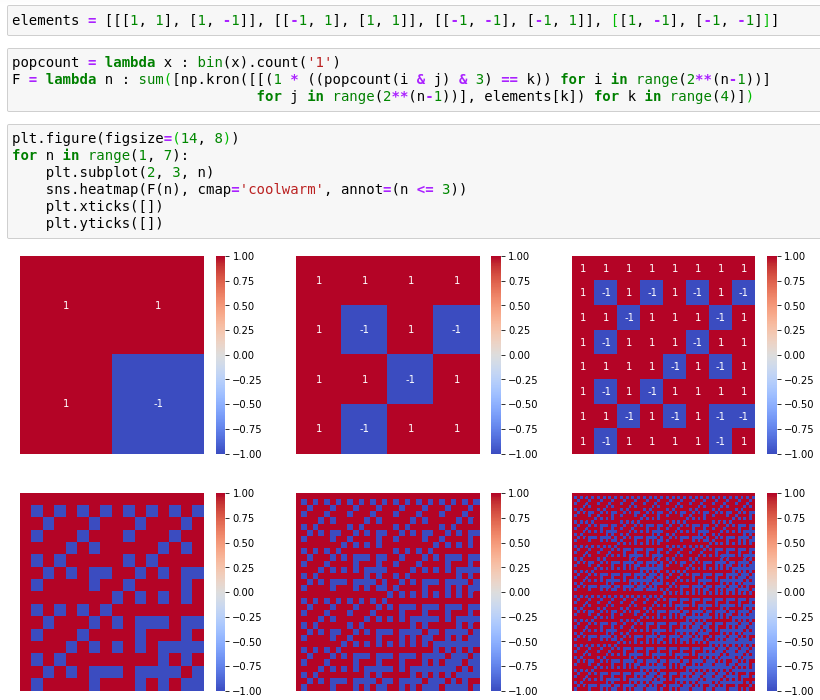The code above uses the following equivalent formulation:

• Take an M-by-M array, where$M = 2^{n-1}$, and number the rows/columns in a 0-indexed fashion.
• Define the weight of the (i, j)th entry to be popcount(i & j). That is to say, we take the size of the intersection between the set of ‘1’-bits in the binary expansions of i and j.
• Populate the (i, j)th entry with a 2-by-2 symmetric matrix according to the weight modulo 4:
• if 0 modulo 4, use the matrix [[1, 1], [1, -1]];
• if 1 modulo 4, use the matrix [[-1, 1], [1, 1]];
• if 2 modulo 4, use the matrix [[-1, -1], [-1, 1]];
• if 3 modulo 4, use the matrix [[1, -1], [-1, -1]].

(These are precisely the four real symmetric 2-by-2 (±1)-matrices which square to 2I.)

Other constructions of the Barnes-Wall lattices appear in:

• Gabriele Nebe, E. M. Rains, and Neil Sloane, A Simple Construction for the Barnes-Wall Lattices (using the ring$\mathbb{Z}[\sqrt{2}]$ ).
• Daniele Micciancio and Antonio Nicolosi, Efficient Bounded Distance Decoders for Barnes-Wall Lattices (using the ring$\mathbb{Z}[i]$ of Gaussian integers).
• Conway and Sloane, Sphere Packings, Lattices and Groups (using Reed-Muller codes).
Posted in Uncategorized | 1 Comment

## Subsumptions of regular polytopes

We say that a regular n-dimensional polytope P subsumes a regular n-dimensional polytope Q if the vertex-set of Q is geometrically similar to a subset of the vertex-set of P.

For instance, the dodecahedron subsumes a cube (the convex hull of the red and blue vertices below), which in turn subsumes a tetrahedron (the convex hull of the blue vertices alone):Note that the centroid of a regular polytope is also the circumcentre — the unique point equidistant from all of the polytope’s vertices — which implies that if one polytope subsumes another, the two vertex-sets must share the same centre. Consequently, we assume without loss of generality that regular polytopes are always centred on the origin.

### Two and three dimensions

The only regular polytopes in two dimensions are the regular polygons. Using the above reasoning, it is straightforward to see that an m-gon subsumes an n-gon if and only if n is a divisor of m.

In three dimensions, there are exactly five regular polytopes, the Platonic solids. We have already seen that the tetrahedron, cube, and dodecahedron form a chain of subsumptions. It can be shown that these are the only subsumptions between Platonic solids. In particular, if we normalise the solid P to be centred on the origin and have unit radius, we can calculate the set S(P) of pairwise inner products between the vertices of the polyhedron. If P subsumes Q, then S(Q) must necessarily be a subset of S(P).

### Four dimensions

In four dimensions, there are six ‘Platonic solids’. Five of these form a subsumption chain:

• the orthoplex (generalised octahedron) is subsumed by
• the hypercube, which is subsumed by
• the 24-cell, which is subsumed by
• the 600-cell, which is subsumed by
• the 120-cell.

There’s a really elegant way to see these inclusions in terms of quaternions. The group Q8 of eight quaternions {±1, ±i, ±j, ±k} form the vertices of an orthoplex.

This is an index-3 subgroup of the binary tetrahedral group, which contains these eight quaternions together with the 16 unit quaternions of the form:

½(±1 ± i ± j ± k)

These 16 quaternions manifestly form the vertices of a hypercube. Moreover, because we can partition the binary tetrahedral group into cosets with respect to the subgroup Q8, it follows that this hypercube is the union of two disjoint orthoplexes. Taken together, these 24 quaternions form the vertices of a 24-cell, a four-dimensional regular polytope which has no analogue in three dimensions.

Greg Egan’s animation below shows the three cosets in red, green, and blue. Each coset forms the vertices of an orthoplex; the union of any two cosets forms the vertices of a hypercube; the union of all three cosets forms the vertices of a 24-cell: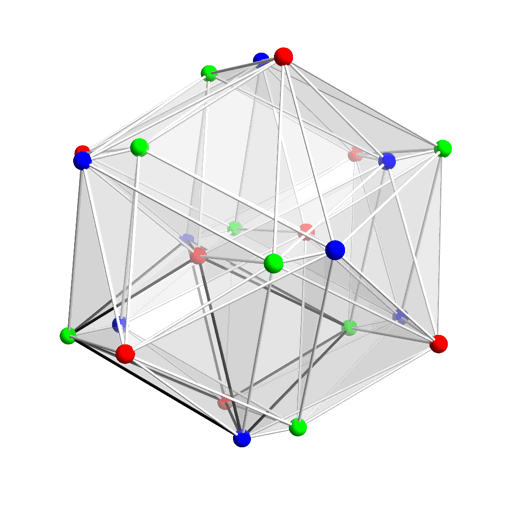Animation by Greg Egan of a 24-cell undergoing a double rotation.

The 600-cell has 120 vertices which can be identified with the unit icosians, a group of 120 unit quaternions which contains the binary tetrahedral group as an index-5 subgroup. It follows, therefore, that the 600-cell subsumes the 24-cell.

If you take the ring generated by the unit icosians, the 600 norm-2 elements form a scaled copy of the 120-cell, a four-dimensional analogue of a dodecahedron. We can identify norm-2 elements if one can be obtained from the other by right-multiplication by a unit icosian; this partitions these 600 vertices into five equivalence classes, each geometrically similar to the 120 vertices of a 600-cell.

The remaining four-dimensional regular polytope, the simplex, is not subsumed by any of these polytopes; again, this can be deduced from looking at the set S of inner products.

### Higher dimensions

In higher dimensions, there are only three regular polytopes: the simplex, the orthoplex, and the hypercube.

S(simplex) is the set {1, −1/n}, and S(orthoplex) is the set {1, 0, −1}. It follows that neither of these can subsume each other. This leaves the question of whether the hypercube can subsume either of the other two regular polytopes. The answer is that it depends on n, and is an unsolved problem!

The orthoplex consists of n orthogonal pairs of opposite vertices. For this to be subsumed by a hypercube is equivalent to the existence of a matrix M consisting entirely of entries ±1 such that H := M / √d is a real orthogonal matrix. Such Hadamard matrices exist whenever n is a power of 2. If n ≥ 3, it is easy to show that n must be a multiple of 4, and the converse is conjectured to be true:

Does a Hadamard matrix exist in dimension n whenever n is divisible by 4?

The first unsolved case is n = 668.

When does the hypercube subsume a simplex? This is equivalent to having n+1 vectors with entries ±1 such that, for every pair of vectors, they disagree in sign in (n+1)/2 coordinates and agree in sign in the other (n−1)/2 coordinates. If we appended an (n+1)th coordinate which is identically 1 to every vector, they would all be orthogonal and therefore form a Hadamard matrix. The converse is also true: removing a column of a Hadamard matrix results in a set of rows which form the vertices of a regular simplex.

To conclude:

• The hypercube subsumes the simplex if and only if there is a Hadamard matrix of dimension n+1;
• The hypercube subsumes the orthoplex if and only if there is a Hadamard matrix of dimension n.
Posted in Uncategorized | 1 Comment

## Relocation

In June of this year, I read Paul Graham’s essay on names. The author (whom you may know from his book On Lisp) begins with the following advice:

If you have a US startup called X and you don’t have x.com, you should probably change your name.

I’d relax the consequent of this advice to:

If you have a US startup called X and you don’t have x.com, you should probably change this situation.

Changing your name from X to something else is one possibility, but acquiring x.com (if possible) is often a better solution. Changing a name is often not a zero-cost operation — the Royal Mail found out the hard way in the early 2000s — so this option should not be taken lightly.

When I’d checked in previous years, hatsya.com was unavailable. When I checked this year, the previous owners must have allowed their registration to elapse; it had been swiftly snapped up by a ‘domainer’: a market-maker which buys elapsed domains and offers them at a substantially higher price. A few moments of cost-benefit analysis were sufficient to conclude that buying the domain was a good idea.

Anyway, today I intended to write a post on Hadamard matrices, only to find that the WordPress editor had undergone a considerable change which (for me at least) renders it less usable than before. The ‘classic editor’ is still available as a plugin, but this plugin is only free for self-hosted websites. This gave me the impetus to switch! As such:

• Complex Projective 4-Space is now self-hosted at cp4space.hatsya.com instead of cp4space.wordpress.com, with the latter redirecting to the former. This applies ‘recursively’ to the whole site, so no existing links to individual posts are broken.
• Catagolue is now primarily located at catagolue.hatsya.com, which has the advantage (over the default Google domain) of being accessible from within mainland China. The other mirrors still continue to work.

On the subject of Catagolue, the quantity of random soups being searched has increased very rapidly as a result of GPU support being added to the search program. At the time of writing, there have been 40 × 10^12 soups searched using CPU-only instances (from 2015 to present), compared with 104 × 10^12 soups searched using GPU-powered instances (from 2019 to present).

This calendar year has seen the emergence of the first natural period-7 objects: three instances of the loafer and (yesterday) a burloaferimeter variant.

## Associative universal gates

The Boolean function NAND is famously universal, in that any Boolean function on n inputs and m outputs can be implemented as a circuit composed entirely of NAND gates. For example, the exclusive-or operation, A XOR B, can be written as:

(A NAND (B NAND B)) NAND (B NAND (A NAND A))

This raises the question: are there any associative universal functions? It is routine to check that none of the 16 two-input Boolean functions are simultaneously associative and universal, but perhaps we can replace the set {true, false} with a larger set such that a function of this form exists?

Even better, can we find a nontrivial finite group G such that any function from G^n to G^m can be implemented as an n-input m-output circuit composed entirely of 2-input ‘multiplication gates’ (which merely multiply the two inputs, viewed as group elements)?

This is not possible if there is a homomorphism from G to [the additive group of] a finite field F: the induced function from F^n to F^m must be linear, and there exist nonlinear functions from F^n to F^m, so certain functions from F^n to F^m are not realisable as a circuit of multiplication gates. It follows that there exist functions from G^n to G^m which are not realisable as a circuit of multiplication gates either.

If the group G is solvable, then it must have a homomorphism to a nontrivial Abelian group (the quotient of G by its commutator subgroup), which in turn has a homomorphism to a nontrivial prime-power cyclic group (by the structure theorem), which is indeed the additive group of a finite field.

Consequently, we can restrict attention to unsolvable groups, the smallest example of which is A_5, the alternating group on five elements.

### Universality of group operation in A_5

Let γ = (1 2 3 4 5) and consider the function:

f(x) = ((x^15 γ)^6 γ^4)^4

Usefully, the image of f contains exactly two elements — γ and the identity — which we’ll call ‘true’ and ‘false’.

Following the idea from the proof of Barrington’s theorem, we can also implement Boolean logic gates. Specifically, the elements γ = (1 2 3 4 5), δ = (1 3 5 4 2), and ε = (1 3 2 5 4) are all conjugate to each other in A_5, and ε is the group commutator of γ and δ. A Boolean AND gate (which expects each input to be either the identity or γ) can be implemented by:

• conjugating one of the inputs by an appropriate constant (so that if it were originally γ, it becomes δ);
• taking the commutator of that with the other input (resulting in ε iff both inputs were γ);
• conjugating the output by an appropriate constant (so that the output is γ rather than ε).

Similarly, a NOT gate can be implemented by multiplying the input by γ^4 and then conjugating by an appropriate double-transposition. This means that we can construct arbitrary Boolean logic circuits out of ‘A_5 composition gates’ together with constants.

Finally, since every element of A_5 can be obtained by multiplying together a bunch of conjugates of γ, then for each pair of elements α, β of A_5 we can create a ‘demultiplexer gate’ which outputs α when the input is γ and outputs β when the input is the identity — this is analogous to the ‘ternary operator’ (x ? α : β) familiar from many programming languages.

Consequently, we can implement an arbitrary function from (A_5)^n to A_5 by doing the following:

• for each of the n inputs, multiply it by each of the 60 elements of A_5 and apply f to each of these 60 results (so for each of the n inputs, we get a 60-bit ‘signature’ which acts as an (inefficient!) injective binary encoding of that input);
• apply some complicated Boolean logic circuit composed of the AND and NOT gates described above, which has 60 Boolean outputs (containing a one-hot encoding of the output of the function);
• apply appropriate demultiplexer gates to the outputs (so that one of these 60 outputs is the desired output, and the other 59 outputs are the identity element) and multiply the results together (in any order!).

An arbitrary function from (A_5)^n to (A_5)^m can then be implemented by m non-interacting single-output circuits of the above form, all sharing the same n inputs.

Note that this is a very inefficient construction, but it suffices to establish the universality of the composition gate for A_5-valued logic. We’ll discuss efficiency more in a later post, when we discuss Barrington’s theorem and applications thereof.

## Another two rational dodecahedra

Since finding one rational dodecahedron inscribed in the unit sphere, I decided to port the search program to CUDA so that it can run on a GPU and thereby search a larger space in a reasonable amount of time. Firstly, let us recall our search methodology: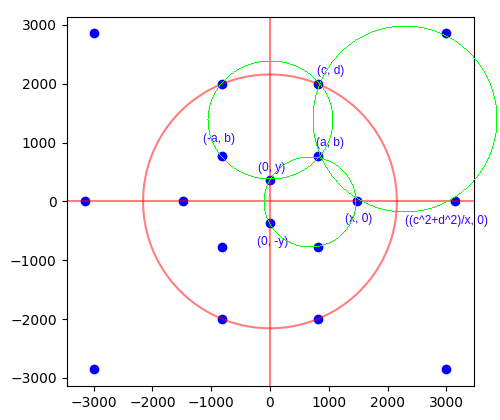We exhaustively look for all small positive integer points (a, b) and (c, d) satisfying b < d and ad > bc. The first of these inequalities ensures that (c, d) is further up the upper-left circle than (a, b); the second inequality ensures that the line through the origin and (a, b) intersects the upper-right circle at two distinct points.

For each such candidate pair of points, we analytically compute x and y, checking that they are rational. If so, we check that the four indicated points on the lower circle are indeed concyclic by performing a final determinant check.

This is embarrassingly parallel, so well-suited for a GPU. We launch one thread for each pair of 1 ≤ a, dN, where N = 10000, so a total of 10^8 threads are launched. Each thread performs two nested loops: the outer loop searches each value of b up to (but excluding) d; the inner loop starts with c = 1 and increments it until the inequality ad > bc is violated or c exceeds the bound N.

### Dealing with integer overflow

One rather annoying bugbear for searching a larger space was that of integer overflow. In the process of computing x, we need to take the square-root of a degree-6 polynomial in the four integer parameters a, b, c, d and check that this square-root is an integer*: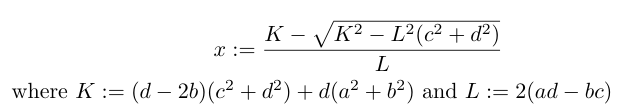*since the expression for x is rational if and only if$\sqrt{K^2 - L^2(c^2 + d^2)}$ is an integer.

When the integer parameters grow beyond 1000, this degree-6 polynomial can easily overflow the maximum representable 64-bit integer. To circumvent this problem, we take the following ugly approach:

• Compute$K^2 - L^2(c^2 + d^2)$ in both double-precision floating-point arithmetic (which is approximate) and in 64-bit integer arithmetic (which is exact, but reduced modulo 2^64).
• ‘Repair’ any loss of relative accuracy (caused by the subtraction) in the double-precision approximation by rounding to the nearest multiple of 2^64 and adding the exact result reduced into the interval [-2^63, 2^63 – 1].
• Compute the double-precision square-root. This will have comparable relative error, and therefore small absolute error (i.e. less than 1).
• Cast the square-root back to a 64-bit integer (which won’t overflow).
• Check this integer and nearby values to see whether they square (modulo 2^64) to the desired result.

Source code: here.

The analogous computation for y involves polynomials of degree at most 4, so we can safely search all a, b, c, d <= 10000 without overflow. The final determinant check is just the evaluation of a polynomial, so we can use arithmetic modulo 2^64 and not worry about integer overflow until later.

### Hardware

I opted to use an NVIDIA Volta V100 because it’s the most powerful GPU to which I currently have access (via Amazon Web Services). The V100 is huge, consisting of 80 streaming multiprocessors, each of which is capable of simultaneously issuing 4 instructions per cycle, where each instruction is vectorised over 32 ‘threads’. (For this reason, the GPU is sometimes advertised as having 80 × 4 × 32 = 5120 ‘CUDA cores’, but these are not comparable with CPU cores; a modern CPU core with vectorisation capabilities and multithreading is more akin to an entire streaming multiprocessor.)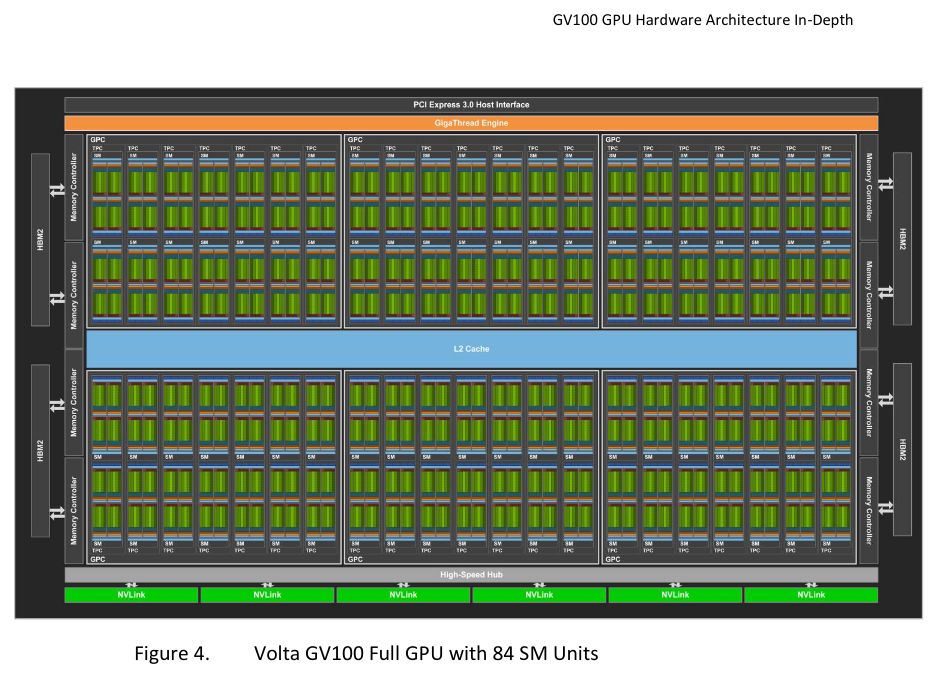Schematic of the Volta V100 from the whitepaper. Only 80 of the 84 streaming multiprocessors are actually ‘activated’; this means that the fabrication of the chip is allowed to contain up to 4 manufacturing defects without affecting the validity of the resulting GPU.

Each of the 80 streaming multiprocessors also has 8 ‘tensor cores’, which perform reduced-precision matrix multiplications useful for neural networks. These tensor cores were unused by this search program, as the nature of this particular problem requires high-precision 64-bit integer and double-precision floating-point computations.

Compiling the program with the switch -Xptxas=-v shows that there is no expensive register spillage or other performance problems:

$nvcc -O3 -Xptxas=-v -lineinfo cudodeca.cu -o cudodeca ptxas info : 56 bytes gmem ptxas info : Compiling entry function '_Z12dodecakernelv' for 'sm_52' ptxas info : Function properties for _Z12dodecakernelv 64 bytes stack frame, 0 bytes spill stores, 0 bytes spill loads ptxas info : Used 47 registers, 320 bytes cmem, 24 bytes cmem Sixteen hours of continual uninterrupted runtime on the V100 (costing a total of about fifty US dollars) were sufficient to exhaust this space and show that there exist at most 3 primitive solutions within these bounds (full output here). The second and third solutions are much larger than the first: Solution 1: a=22, b=21, c=22, d=54, x=40, y=10 Solution 2: a=1680, b=1474, c=2023, d=2601, x=2890, y=578 Solution 3: a=2860, b=3915, c=3297, d=6594, x=7065, y=2355  The reason for saying ‘at most 3 primitive solutions’ is that the final determinant check is performed modulo 2^64, so false positives can slip through. To be absolutely sure of these results, we need an additional manual verification step using unbounded (‘bigint’) integer arithmetic. Unbounded integers are natively provided in languages such as Python, Haskell, and Wolfram Mathematica; we use the latter for convenience. ### Verification We can check the three determinants for the putative solution (1680, 1474, 2023, 2601, 2890, 578)… $ wolfram12.0.0
Mathematica 12.0.0 Kernel for Linux x86 (64-bit)

In:= f = Function[{x, y}, {1, x, y, x^2 + y^2}]

Out= Function[{x, y}, {1, x, y, x^2 + y^2}]

In:= Det[{f[a, b], f[x, 0], f[0, y], f[0, -y]}] /. {a -> 1680, b -> 1474, c -> 2023, d -> 2601, x -> 2890, y -> 578}

Out= 0

In:= Det[{f[(c^2+d^2)/x, 0], f[c, d], f[a, b], f[x, 0]}] /. {a -> 1680, b -> 1474, c -> 2023, d -> 2601, x -> 2890, y -> 578}

Out= 0

In:= Det[{f[c, d], f[a, b], f[-a, b], f[0, y]}] /. {a -> 1680, b -> 1474, c -> 2023, d -> 2601, x -> 2890, y -> 578}

Out= 0

…and likewise for the putative solution (2860, 3915, 3297, 6594, 7065, 2355)…

In:= Det[{f[a, b], f[x, 0], f[0, y], f[0, -y]}] /. {a -> 2860, b -> 3915, c -> 3297, d -> 6594, x -> 7065, y -> 2355}

Out= 0

In:= Det[{f[c, d], f[a, b], f[-a, b], f[0, y]}] /. {a -> 2860, b -> 3915, c -> 3297, d -> 6594, x -> 7065, y -> 2355}

Out= 0

In:= Det[{f[(c^2+d^2)/x, 0], f[c, d], f[a, b], f[x, 0]}] /. {a -> 2860, b -> 3915, c -> 3297, d -> 6594, x -> 7065, y -> 2355}

Out= 0

…confirming that these are indeed valid primitive solutions.

Posted in Uncategorized | 4 Comments

## Banach-Tarski and the Axiom of Choice

Tomasz Kania and I recently coauthored a paper about Banach spaces. The paper makes extensive use of the axiom of choice, involving a transfinite induction in the proof of Theorem B as well as several appeals to the fact that every vector space admits a Hamel basis.

The axiom of choice is often misunderstood, as is many of its consequences. I often hear the Banach-Tarski ‘paradox’ being quoted as a philosophical argument against the truth of the axiom of choice. However, the statement of the Banach-Tarski theorem is not paradoxical at all. One way to state Banach-Tarski is:

• The set of points in a closed unit ball can be rearranged into the disjoint union of two copies of the set of points in a closed unit ball through a finite sequence of operations of splitting a set [into a disjoint union of two subsets], rotating a set, translating a set, and recombining two disjoint sets into their union.

Note that the set of points in a closed unit ball is an [uncountably] infinite set. We are perfectly accustomed to the points in an infinite set being in bijective correspondence with two copies of the original set: for instance, the even integers and the odd integers are each isomorphic to the integers. So we could write the following uncontroversial statement which differs from the Banach-Tarski theorem in only the indicated locations:

• The set of integers can be rearranged into the disjoint union of two copies of the set of integers through a finite sequence of operations of splitting a set [into a disjoint union of two subsets], applying an affine transformation to a set, and recombining two disjoint sets into their union.

In particular, we split the integers into the odd integers and the even integers, and affine-transform each of these sets into a copy of all of the integers. This is uncontroversial, and doesn’t require the axiom of choice. No-one would non-ironically argue that this implies the arithmetic statement 1 = 1 + 1, because it is intuitively obvious that the set of integers is infinite and that the only statement about cardinals that this immediately implies is that ℵ_0 = ℵ_0 + ℵ_0.

However, people often feel differently about the Banach-Tarski ‘paradox’ because a closed unit ball feels like a tangible solid object. It is often joked that the Banach-Tarski paradox can be used to duplicate approximately-spherical real-world objects, such as oranges, as was the subject of this rather bizarre music video:

Notwithstanding any physical intuition, a closed unit ball is nonetheless an uncountable set of points. The axiom of choice merely gives us extra freedom in manipulating certain infinite sets and is particularly useful for constructions that involve induction over uncountable sets.

The Banach-Tarski theorem is still mathematically interesting and nontrivial, unlike the statement we made about rearranging the integers. It proves that there is no translation-invariant rotation-invariant finitely-additive measure on$\mathbb{R}^n$ (n >= 3), whereas such a measure does exist in n = 2 dimensions as proved by Banach.

The proof of Banach-Tarski is even more interesting than the statement, and is well worth reading. It relies on the fact that the free group on two generators can be embedded as a subgroup of SO(n) when n >= 3; this is not the case for n = 2.

But what Banach-Tarski certainly does not imply is this nonsense*: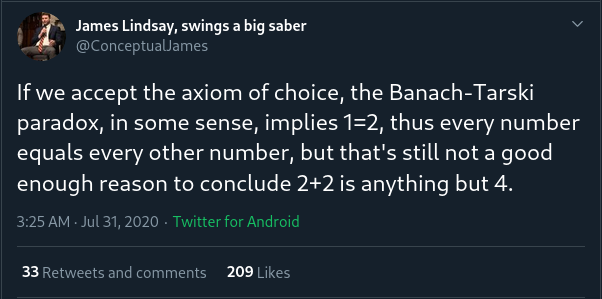A misunderstanding of Banach-Tarski

(*ordinarily I would be more polite when someone is wrong on the internet, but the author of this tweet has been engaging in a highly dubious trolling campaign. Tim Gowers has weighed in on the discussion with an informative thread.)

### The constructible universe

There’s actually a more fundamental reason why the axiom of choice cannot possibly be blamed for any results in arithmetic, false or otherwise. Assuming ZF set theory, then inside the von Neumann universe V is a subclass** (which may or may not be the whole of V) called L, also known as Kurt Gödel’s constructible universe.

**like a subset, but too big to be a set in the set-theoretic sense of the word set.

L is very well-behaved. Firstly, it is an internally consistent model of ZF set theory. Moreover, this operation of taking the constructible universe is idempotent: if we take the constructible universe within L (instead of within V), we still get the whole of L. This means that L is a model of V=L, together with anything that logically follows from V=L such as the axiom of choice or the Generalised Continuum Hypothesis. That is to say that L unconditionally satisfies the axiom of choice even if the full universe V does not.

Finally, and importantly for us, the Shoenfield absoluteness theorem states that certain statements (namely those that are at most Σ^1_2 or Π^1_2 in the analytical hierarchy, which subsumes all statements in first-order Peano arithmetic) are true in V if and only if they are true in L.

In particular, if a statement about first-order Peano arithmetic is proved in ZFC, then the result is also true in ZF (because you can ‘run the proof inside L’ where the axiom of choice holds, and then use the Shoenfield absoluteness theorem to transfer the result back to V). Indeed, you can also freely assume anything else that follows from V=L, such as the truth of the Generalised Continuum Hypothesis. This meant that reliance on the axiom of choice could easily be removed from Wiles’s proof of FLT, for instance.

If the author of that tweet or anyone else managed to prove 2+2=5 using ZFC, then the proof could be modified to also operate in ZF without requiring choice. This would, of course, mean that ZF is inconsistent and mathematics would reënter a state of foundational crisis.

Anyway, this is something of a distraction from the main purpose of this post, which is to briefly discuss one of the many*** useful applications of the axiom of choice.

***other applications include proving Tychonoff’s theorem in topology, the compactness theorem for first-order logic, the existence of nontrivial ultrafilters, that every vector space has a Hamel basis, et cetera.

### Transfinite induction

One equivalent form of the axiom of choice is that every set can be bijected with an ordinal. Ordinals have the property that every non-empty subset of an ordinal has a least element, which makes them ideal for inductive proofs: if you want to prove that a property P holds for all elements, you need only show that there isn’t a least counterexample.

An application of this is to be able to perform a step-by-step construction involving uncountably many ‘steps’. For example, a fun question is:

Can three-dimensional space be expressed as a union of disjoint circles?

Using a transfinite induction, it is possible to place each of the uncountably many circles one at a time, avoiding any previous circles and ensuring that every point in the ambient space has been accounted for. Peter Komjath described such a construction in an answer to the question when it was asked on MathOverflow: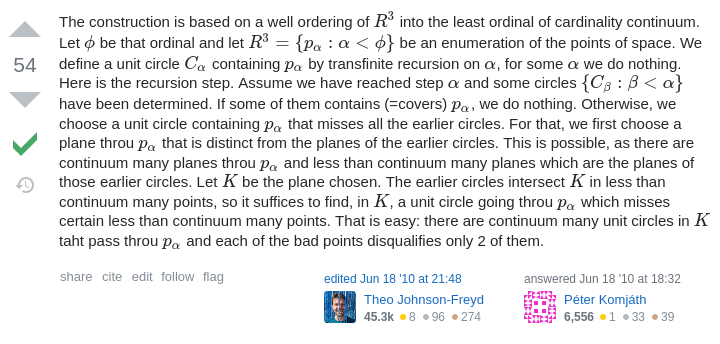It is worth emphasising that this uses the least ordinal of cardinality continuum. These ‘initial ordinals’ have the useful property that all previous ordinals are strictly smaller from a cardinality perspective. This means that at each stage in the transfinite induction, the number of circles that have already been emplaced is strictly lower than the cardinality of the continuum, so there’s plenty of room to insert another circle passing through a specified point. This same idea was used in the paper I coauthored with Tomasz Kania.

A generalisation of this problem which remains open is whether there exists such a partition where every pair of circles is pairwise linked. The Hopf fibration provides a solution where$\mathbb{R}^3$ is augmented with an extra point at infinity, where every pair of circles interlock in the same manner as these two rings of dodecahedra:Without this point at infinity, though, the problem is much harder and has evaded solution. Transfinite induction can show that we can cover all but one point with disjoint linked circles, but there is no easy way to modify the proof to cover the last point.

## Rational dodecahedron inscribed in unit sphere

Moritz Firsching asked in 2016 whether there exists a dodecahedron, combinatorially equivalent to a regular dodecahedron, with rational vertices lying on the unit sphere. The difficulty arises from the combination of three constraints:

1. The twelve pentagonal faces must all be planar;
2. The vertices must all be rational points;
3. The vertices must all lie on the unit sphere.

Conditions 1 and 2 are realised by Schulz’s dodecahedron (shown in Firsching’s question). Conditions 1 and 3 are realised by a regular dodecahedron. But finding a solution that satisfies all three conditions simultaneously turns out to be hard. A computer-assisted search only found a single example, up to Möbius transformations of the unit sphere: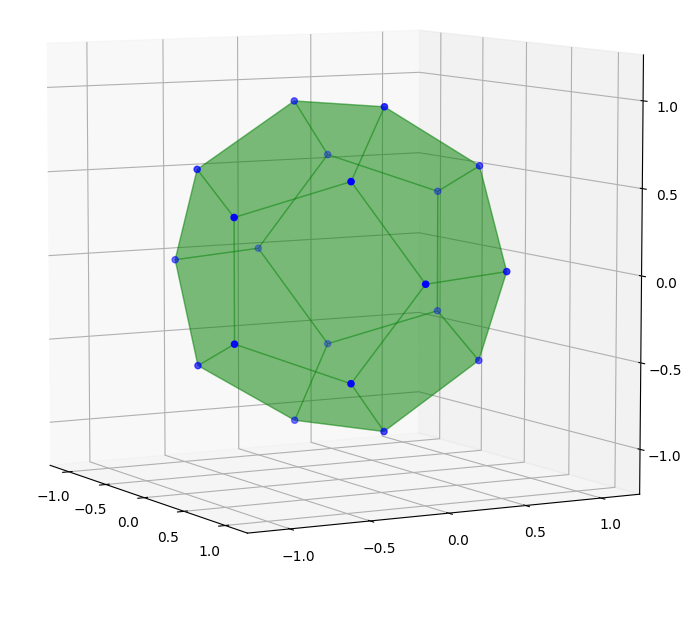The vertices are obtained from the following set of integer lattice points:

iverts = [[ 1840720, 1798335, 0],
[ 2573375, 0, 0],
[ 773500, 1963500, -1472625],
[ 773500, 1963500, 1472625],
[ 794070, 323510, -2426325],
[ 794070, 323510, 2426325],
[ 905828, -2223396, -926415],
[ 905828, -2223396, 926415],
[ 1925420, -863940, -1472625],
[ 1925420, -863940, 1472625],
[-1840720, -1798335, 0],
[-2573375, 0, 0],
[ -773500, -1963500, 1472625],
[ -773500, -1963500, -1472625],
[ -794070, -323510, 2426325],
[ -794070, -323510, -2426325],
[ -905828, 2223396, 926415],
[ -905828, 2223396, -926415],
[-1925420, 863940, 1472625],
[-1925420, 863940, -1472625]]

by dividing everything by 2573375. The planarity of the pentagonal faces and the fact that the points lie on a radius-2573375 sphere are checked by this notebook.

The dodecahedron has an order-8 symmetry group (it has three orthogonal planes of reflectional symmetry) and is visually very close to being regular. The symmetry group was a consequence of the search methodology; I specifically looked for solutions with this symmetry group in order to narrow down the search space.

By polar reciprocation, this also answers the equivalent question: ‘does there exist an icosahedron with rational vertices that contains an inscribed unit sphere?’

### Methodology

Subject to the points being on the unit sphere, the planarity is equivalent to concyclicity. After stereographically projecting the unit sphere to the plane, which preserves rationality of points, the problem becomes:

Does there exist an embedding of the dodecahedral graph into the plane, with each vertex being a rational point, such that the five vertices of each face are all concyclic?

I restricted attention to symmetrical solutions invariant under reflection in each of the coordinate axes and under inversion in a circle centred on the origin:(Note that the two points furthest from the origin are not shown in the above diagram.)

Here the red lines and circle are the axes of reflection and inversion, respectively. The three green circles are the necessary concyclicities, implying that the other nine pentagons are also concyclic by symmetry. These can be translated into Diophantine equations by using the fact that the four points {(x1, y1), (x2, y2), (x3, y3), (x4, y4)} are concyclic or collinear if and only if the following 4-by-4 determinant vanishes:

• 1, x1, y1, x1² + y1²;
• 1, x2, y2, x2² + y2²;
• 1, x3, y3, x3² + y3²;
• 1, x4, y4, x4² + y4²;

I wrote a program to search over integer values of a,b,c,d and check whether the values of x and y implied by the upper-right and upper-left green circles, respectively, are rational. If so, the program proceeds to check that the remaining (lower) green circle holds; if so, it reports a solution. The program reported several solutions, but they were all scalar multiples of a single ‘primitive’ solution and therefore correspond to the same polyhedron.

### Do there exist any more solutions?

My search program (available here) only found a single primitive solution, even when the threshold was increased to N = 1000. This is not particularly conclusive: it’s often the case that Diophantine equations have solutions that are very few and far between, even if there are infinitely many solutions; the Pell equation is a simple example of this phenomenon.

EDIT: after running on a GPU with N = 10000, another two primitive solutions emerged.

Of course, if history is any guide, it’s entirely likely that Noam Elkies will storm in at any moment wearing a superhero cape and brandishing an infinite family of solutions to this problem.

Posted in Uncategorized | 4 Comments

## Fast-growing functions revisited

There have been many exciting results proved by members of the Googology wiki, a website concerned with fast-growing functions. Some of the highlights include:

• Wythagoras’s construction of an 18-state Turing machine which takes more than Graham’s number of steps to terminate.
• LittlePeng9’s construction of a Turing machine which simulates the Buchholz hydra, showing that ‘BB(160,7)>>most of defined computable numbers’.

Recently, however, there have been claims that several of the results on cp4space pertaining to fast-growing functions have never been proved. For the record, here are the original (hitherto unpublished) proofs with all of the missing details filled in such that they are no longer ‘exercises for the reader’:

### Theorem 1: TREE(3) > f^5(8), where f(n) := tree^n(7)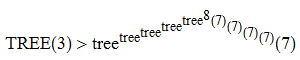To prove this (see here for definitions), it is sufficient to exhibit a sequence of trees whose nodes are each coloured red, green, or blue, such that there is no colour-preserving embedding of an earlier tree into a later tree. We begin the sequence as follows: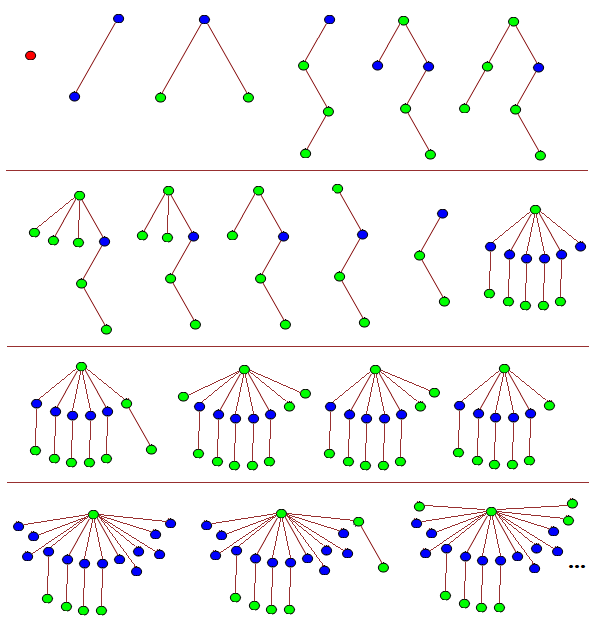The first tree is a single red node, and we henceforth have no red nodes in any of the later trees. The twelfth tree, T12, and all subsequent trees have a single green root node connected to:

• n branches containing a single blue node attached to a single green leaf;
• m branches containing a single blue leaf;
• one or more green trees.

Trees T1 through to T11 can be seen to not embed into any tree of this form, so it remains to define the sequence {T12, ….} and show that none of these trees embeds into any later tree.

The sequence begins as follows:

T1 {}
T2 [[]]
T3 [()()]
T4 [((()))]
T5 ([(())][])
T6 ([(())](()))
T7 ([(())]()()())
T8 ([(())]()())
T9 ([(())]())
T10 ([(())])
T11 [(())]
T12 ([()][()][()][()][()][])
T13 ([()][()][()][()][()](()))
T14 ([()][()][()][()][()]()()())
T15 ([()][()][()][()][()]()())
T16 ([()][()][()][()][()]())
T17 ([()][()][()][()][()])
T18 ([()][()][()][()][][][][][][][][][])
T19 ([()][()][()][()][][][][][][][][](()))
T20 ([()][()][()][()][][][][][][][][]()()())
T21 ([()][()][()][()][][][][][][][][]()())
T22 ([()][()][()][()][][][][][][][][]())
T23 ([()][()][()][()][][][][][][][][])
T24 ([()][()][()][()][][][][][][][]X7)

where X7 is any (monochromatically green) tree on 7 vertices. This can be followed by the sequence:

T25 ([()][()][()][()][][][][][][][]X8)
T26 ([()][()][()][()][][][][][][][]X9)
T27 ([()][()][()][()][][][][][][][]X10)
...
T_(23 + tree(7)) ([()][()][()][()][][][][][][][]())

where X7, X8, X9, X10, … () is a maximal-length sequence for tree(7). If any earlier tree here embeds into a later tree, then the same must have been true in the maximal-length sequence for tree(7), contradicting the definition.

Consequently, we’ve already proved that TREE(3) > tree(7). We can then extend the sequence further by ‘burning’ another blue node (pair of square brackets):

T_(24 + tree(7)) ([()][()][()][()][][][][][][][])
T_(25 + tree(7)) ([()][()][()][()][][][][][][]Y1)

where Y1 is the first tree in the maximal-length sequence for tree(tree(7)). We proceed in the same way as before with a sequence of tree(tree(7)) terms, culminating in:

T_(23 + tree(7) + tree(tree(7))) ([()][()][()][()][][][][][][]())
T_(24 + tree(7) + tree(tree(7))) ([()][()][()][()][][][][][][])
T_(25 + tree(7) + tree(tree(7))) ([()][()][()][()][][][][][]Y2)

where Y2 is the first tree in the maximal-length sequence for tree(tree(tree(7))). Repeating this process, we eventually reach the tree:

([()][()][()][()])

at time 24 + tree(7) + tree(tree(7)) + … + tree^8(7). We can then make the next tree in our process have the form:

([()][()][()][][][][][]...[]X7)

where we have created tree^8(7) new blue nodes by ‘burning’ a branch of the form [()]. The same argument as before allows us to reach the tree:

([()][()][()])

at time well beyond tree^(tree^8(7))(7). Repeating this outer iteration another three times gets us to the claimed bound.

To see that no tree embeds homeomorphically into a later tree, we note that if T precedes T’, then we have at least one of the following:

• T contains more copies of [()] than T’;
• T contains more copies of [] than T’;
• The monochromatic green subtree of T precedes (in some tree(k) sequence) the monochromatic green subtree of T’;

where the latter condition was ensured by using a sequence for tree(k) which has this property by definition.

### Theorem 2: tree(n) outgrows f_alpha(n) for every alpha preceding the small Veblen ordinal

This is implied by the statement in Harvey Friedman’s e-mails to the FOM mailing list:

One of our many finite forms of Kruskal's theorem asserts that

for all k, TREE[k] exists.

This function eventually dominates every provably recursive
function of the system ACA_0 + Pi12-BI.

and this is elaborated upon in Deedlit’s excellent MathOverflow answer.

### Theorem 3: SSCG(4n + 3) >= SCG(n)

Conveniently, someone had e-mailed me to ask for an explicit proof of this statement, so I’ll include the reply I sent:

Suppose you have a sequence of N = SCG(n) (not necessarily simple)
subcubic graphs, (G_1, ..., G_N), such that G_i has at most i + n
vertices and no graph is a minor of a later graph.

Now, we let H_i be obtained from G_i by replacing each vertex with
a copy of the 4-vertex 3-edge Y-shaped graph, K3,1. For each pair
of adjacent vertices in the original graph, we join two as-yet-unused
'limbs' of the corresponding Y-shapes in the new graph. Then H_i is
simple and subcubic, and we can show that no graph is a minor of a
later graph. This is most easily shown by the fact that for subcubic
graphs, 'minor' is equivalent to 'topological minor', so it suffices
to show that no graph is homeomorphically embeddable into a later
graph. (Proof: if H_i embeds into H_j, the degree-3 vertices of H_i
must map to degree-3 vertices of H_j; this induces a homeomorphic
embedding from G_i to G_j.)

At this point we're almost done. The graphs (H_1, H_2, H_3, ...)
have at most (4n + 4, 4n + 8, 4n + 12, ...) vertices, respectively.
To prove the claim, we actually want a sequence with at most
(4n + 4, 4n + 5, 4n + 6, ...).

Now, since G_1 contains at least 2 vertices, it must contain at
least 1 edge which is not a self-loop (otherwise, every vertex has
degree <= 2 and we can add another edge, producing a more optimal
initial graph G_0, contradicting the assumption that our original
sequence of graphs is maximal). Consequently, H_1 contains a path
of vertices of degrees (3, 2, 2, 3). Let H_1' be the graph obtained
by contracting this path once, and H_1'' be the graph obtained by
contracting it again. Then the graphs (H_1, H_1', H_1'', H_2, H_3,
...) have the property that no graph is a minor of a later graph.

Hence, we can construct the sequence which begins:

- H_1 with at most 4n + 4 vertices;
- (H_1' + K_2) with at most 4n + 5 vertices;
- (H_1' + K_1 + K_1) with at most 4n + 6 vertices;
- (H_1' + K_1) with at most 4n + 7 vertices;
- H_1' with at most 4n + 8 vertices;
- (H_1'' + C_7) with at most 4n + 9 vertices;
- (H_1'' + C_6) with at most 4n + 10 vertices;
- (H_1'' + C_5) with at most 4n + 11 vertices;

where + denotes disjoint union of graphs. At this point, we've
'bought ourselves enough vertices' to continue the sequence as
follows:

- (H_2 + K_1 + K_1 + K_1 + K_1) with at most 4n + 12 vertices;
- (H_2 + K_1 + K_1 + K_1) with at most 4n + 13 vertices;
- (H_2 + K_1 + K_1) with at most 4n + 14 vertices;
- (H_2 + K_1) with at most 4n + 15 vertices;

- (H_3 + K_1 + K_1 + K_1 + K_1) with at most 4n + 16 vertices;
- (H_3 + K_1 + K_1 + K_1) with at most 4n + 17 vertices;
- (H_3 + K_1 + K_1) with at most 4n + 18 vertices;
- (H_3 + K_1) with at most 4n + 19 vertices;

and so forth. The property of no graph being a minor of a later
graph can easily be seen to be true, because at each iteration
we either have:

- the 'big component' (the union of connected components which
contain at least one degree-3 vertex) gets strictly smaller (in
the poset of graphs under the minorship relation);
- or the 'big component' stays identical and the 'small
component' gets strictly smaller;

and any homeomorphic embedding of one graph into another must map
the big component of the first graph into the big component of the
other graph.

I'm sure that there's enough slack in this proof to improve the
upper bound to SSCG(4n + 2) (by starting with H_1' instead of H_1),
but this proof suffices to show the claimed statement.

### Theorem 4: SSCG(3) > TREE^(TREE^2(3))(3)

Again, we want to exhibit a sequence for SSCG(3) which exceeds TREE^(TREE^2(3))(3). We can begin as follows: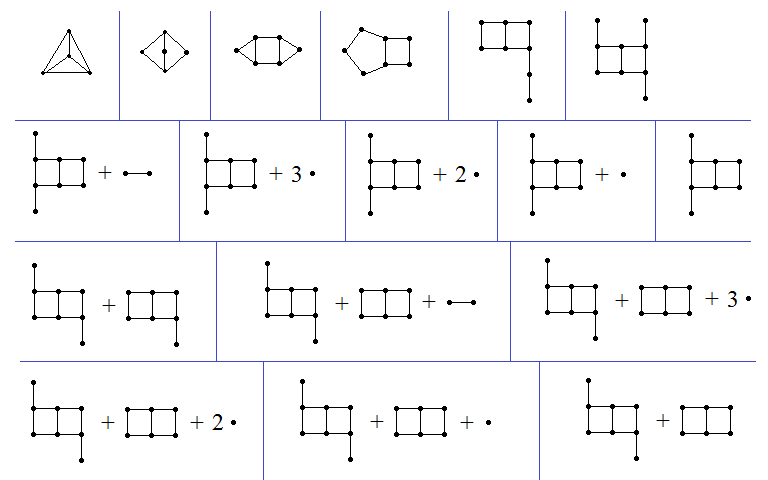All subsequent graphs are the disjoint union of:

• p copies of the double-square with leaves attached to two diametrically opposite degree-2 vertices;
• q copies of the double-square with leaves attached to two degree-2 vertices which are neither adjacent nor diametrically opposite;
• r copies of the double-square with a single leaf;
• s copies of the double-square;
• a graph T which does not contain the double-square as a graph minor.

For example, the last graph in the above sequence is (1, 0, 0, 1, Ø), where Ø is the graph with no vertices or edges.

We define a sequence such that if the graph (p, q, r, s, T) precedes the graph (p’, q’, r’, s’, T’) in the sequence we define, then we have one of the following:

• p > p’;
• p = p’ and q > q’;
• (p, q) = (p’, q’) and r > r’;
• (p, q, r) = (p’, q’, r’) and s > s’;
• (p, q, r, s) = (p’, q’, r’, s’) and T is not a graph minor of T’.

With this convenient notation, we can write down some more terms of the sequence:

G18 (1, 0, 0, 0, 15-cycle)
G19 (1, 0, 0, 0, 14-cycle)
...
G29 (1, 0, 0, 0, square)
G30 (1, 0, 0, 0, triangle = H1)
G31 (1, 0, 0, 0, H2)
G32 (1, 0, 0, 0, H3)
...
G(2^(3 × 2^95) + 20) (1, 0, 0, 0, isolated_vertex)
G(2^(3 × 2^95) + 21) (1, 0, 0, 0, Ø)

where H1, H2, H3, … is the sequence of length 2^(3 × 2^95) − 9 that provides a lower bound for SSCG(2).

We can encode a rooted ordered coloured tree as a graph using the construction described here; this graph satisfies the properties required of T. The long initial segment of length 2^(3 × 2^95) + 21 means that we can use lots of vertices in the next step:

G(2^(3 × 2^95) + 22) (0, 2^(2^96), 0, 0, T1)

where T1 is the encoding of the first tree in a maximal-length sequence of rooted coloured trees for TREE(3). The ‘shrinking counter’ mechanism allows us to obtain a sequence of (l+7) TREE(3) graphs (none of which is a minor of any later graph) culminating in:

G(2^(3 × 2^95) + 22 + (l+7) TREE(3)) (0, 2^(2^96), 0, 0, Ø)
G(2^(3 × 2^95) + 23 + (l+7) TREE(3)) (0, 2^(2^96)-1, TREE(3), 0, U1)

where U1 is the encoding of the first tree in a maximal-length sequence of rooted coloured trees for TREE^2(3). At the end of the graph sequence corresponding to this tree sequence, we have the graph represented by the 5-tuple:

(0, 2^(2^96)-1, TREE(3), 0, Ø)

which can then be followed by:

(0, 2^(2^96)-1, TREE(3)-1, TREE^2(3), V1)

where V1 is the encoding of the first tree in a maximal-length sequence of rooted coloured trees for TREE^3(3). We can continue to repeat this same argument a total of TREE^2(3) times, decrementing the penultimate element of this 5-tuple each time, with the final iteration exceeding TREE^(TREE^2(3))(3) steps before we reach:

(0, 2^(2^96)-1, TREE(3)-1, 0, Ø)

This already proves what we set out to show, but it is clear that the iteration can go much further.

Posted in Fast-growing functions | 7 Comments